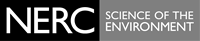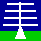THE NERC MST RADAR FACILITY AT ABERYSTWYTH

# Format of MST radar IQ data files

However, users are encouraged to make use of Version-2 MST radar data products.

File contents
These files contain (pre-spectral) in-phase (I) and quadrature (Q) radar return samples.

File naming convention:
iqYYMMDD_hhmm.ddYYis a 2-digit year [00 - 99] MM is a 2-digit month [01 - 12] DD is a 2-digit day [01 - 31] hh is a 2-digit hour [00 - 23] DD is a 2-digit minute [00 - 59] dd is the integer number of minutes covered by the file

Archiving convention: YYYY

File availability
IQ data are not typically recorded and so files exist only when they the data have been stored as part of a special campaign.

File format
The data are stored in non-standard format binary files, as described below:

```			IQ DATA FILES
--------------

File name :-  IQyymmdd_hhmm.min

RANDOM ACCESS FILE  -  UNFORMATTED
RECORD = 64 BYTES

File layout.
------------

Dwell 1,Cycle 1		  Dwell 2,Cycle 1
PB+IQD,IQD,IQD,.....IQD,PB+IQD,IQD,IQD,IQD,.....etc...

Dwell x,Cycle y
....,IQD,IQD,PB+IQD,IQD,IQD,IQD,.....IQD,IQD,BREC

PARAMETER BLOCK ( PB ) ( Start of Each Dwell )
----------------------

Uses 48 bytes out of 64 available.
Following bytes are data values.
NB !! PB allways at the start of a record.

B = BYTE , I2 = INTEGER*2, I4 = INTEGER*4

LTX	B	Length of Transmitter pulse ( uSec ).
NCC	B	Pulse Code Resolution.
0 = Uncoded , 1 = 8 uS , 2 = 4 uS
3 = 2 uS , 4 = 1 uS
IPI	I2	Pulse Repetion Interval ( uSec ).
NPP	I2	Number of Coherent Pulse Additions.
LFT	I2	Length of FFT.
NAV	I2	Number of FFTs Averaged together.
NH1	I2	Start of First Height Range ( Bin No. ).
NH2	I2	End of First Height Range ( Bin No. ).
NBM	I2	Beam Number.
IY	I2	YEAR in Form 90,91,92 etc.
IMN	I2	MONTH ( 0 - 12 )
ID	I2	DAY ( 0 - 31 )
IH	I2	HOUR ( 0 - 23 )	     ]
IM	I2	MINUTE ( 0 - 59 )    ] - Dwell Start time
IS	I2	SECONDS ( 0 - 59 )   ]
NH3	I2	Start of Second Height Range ( Bin No. ).
NH4	I2	End of Second Height Range ( Bin No. ).
NHI	I2	Height Interval in Bins.
NRX	B	Receiver Bandwidth ( uSec ).
DMP	B	Raw Data Collection Flag.
Negative value if Raw Data File Collected.
NDW	I2	Dwell Number.
NCY	I2	Cycle Number.
MST	I2	Run Number.
NRS	I2	Number right shifts in input data.
NXR     I4      Record number of next PB.

IQ DATA ( IQD )
-----------------------

Inphase (I) and Quadrature (Q) data required for
Doppler spectra calculation.
This data is stored as sets of 16 compressed integer
values. Compression is achieved by only using the
number of bits required to store the range of integers
in the 16 value set.
The first 4 bits of the set give the number of bits
minus one used to store each data value.
The values are unsigned so that an offset has to
subtracted from the number to get the true value.

If n is the 4 bit value then the value of the number
is :-  value - (2^n).
Using n+1 bits to extract the value.

The data is stored as all the I samples for the first
height bin followed by all the Q samples, then the second
height bin and so on. Upper height IQ data immediately
follows lower height IQ data.

BLANK RECORD ( BREC )
------------------------

EOF 32 * I2	Zeros

DATA IN FILE
------------

When data is aquired on the MST Radar system a setup file
is used to store the data collection parameters.

The radar system uses the information in this setup file to set
the radar parameters for each beam before it starts to collect
data on that beam.

This information is copied to the parameter block for that beam.
Data is collected on a given beam direction for long enough
to collect all the data points required for the FFT length and
FFT bandwidth specified in the setup file.
FFT bandwidth is :-  1E6 / (IPI * NPP)  ( Hz ).

Up to 20 beams may be used when collecting data and the data
for each beam is called a Dwell.

Data collected for a set of beams is called a Cycle and usually
there will be more than one Cycle in an Observation.
Therefore each beam collected is labelled with its Dwell and
Cycle number.

The first height range refers to data collected below 30 Km
and the second height range is for data collected above 58 Km.
Height of data collected is referenced by range bin which are
at intervals of 150 metres.

To obtain the true height of a given bin an offset must be
subtracted to correct for various time delays in the radar.

IQ Data is obtained from the preprocessor data.
The preprocessor data consists of two sets of inphase and
quadrature data, collected from the two phase detector outputs

If coding was used the first set contains the coded data
and the second set the complementary coded data.

The aquisition programme decodes this data if coding was used
and sums it if not.

The data may be right shifted to prevent overflow depending on
the pulse repetion interval and length of code used.
Additional summing of the data takes place if the FFT
bandwidth required is less than 12 Hz, this also may require
the data to right shifted.

This data, which can now be used to compute the Doppler spectra
for the bins collected, is saved in this file as 'raw' data.
Doppler spectra are 64,128,256 or 512 points in length
and may also be incoherently averaged.

The data is stored as I then Q with height for the first time
sample followed by I & Q with height for the second time sample
and so on until the last time sample.

Upper height IQ data immediately follows lower height IQ data.
The total amount of IQ data saved for each Dwell is :-
16 * (1 + INT((Lower Bins + Upper Bins - 1) / 16))  Bins.
( i.e. sets of 16 height bins ).

```